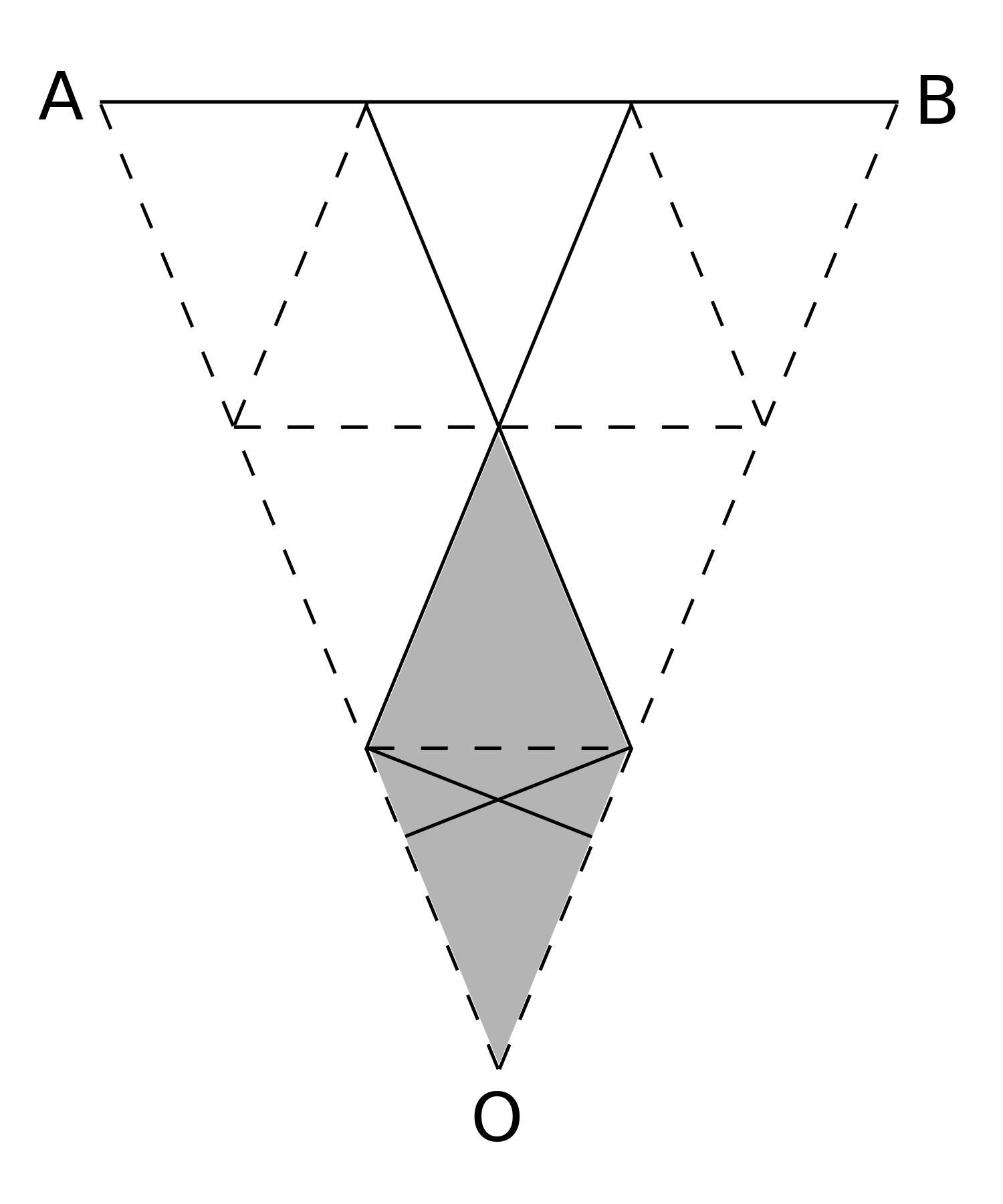#### You may also like### Some(?) of the Parts

A circle touches the lines OA, OB and AB where OA and OB are perpendicular. Show that the diameter of the circle is equal to the perimeter of the triangle### Polycircles

Show that for any triangle it is always possible to construct 3 touching circles with centres at the vertices. Is it possible to construct touching circles centred at the vertices of any polygon?### Circumspection

M is any point on the line AB. Squares of side length AM and MB are constructed and their circumcircles intersect at P (and M). Prove that the lines AD and BE produced pass through P.

# Gaudi's Design

##### Age 11 to 16Challenge Level

The diagram shows part of the ceramic. $A$ and $B$ are vertices of the outer octagon, which has $O$ at its centre. The lines $OA$, $OB$, two lines which are parallel to $AB$ and lines parallel to $OA$ and $OB$ respectively have been added. As can be seen, these lines divide $\triangle OAB$ into nine congruent triangles.

The shaded portion of triangle has area equal to that of two of the smaller triangles. So $\frac{2}{9}$ of the area of $\triangle OAB$ has been shaded. Now, the area of the outer octagon is eight times the area of $\triangle OAB$ and the area of shaded portion of the design is eight times the area of the shaded portion of $\triangle OAB$. Therefore, the fraction of the octagon which is shaded is also $\frac{2}{9}$.